Mobile QR Code1. (Department of Physics, Jeonbuk National University)

Phonon, band splitting, strain, GaAsN

## I. INTRODUCTION

The GaAs$_{\mathrm{1-x}}$N$_{\mathrm{x}}$ epilayer is of considerable importance today, because of its wide application in the optoelectronics device (1-3), and there have been many studies of its physical properties (4-6). Such studies are important because a proper understanding of the material characteristics underlies all applications. It is well known that the GaAs$_{\mathrm{1-x}}$N$_{\mathrm{x}}$ epilayer is grown as a pseudomorphic layer on top of a GaAs buffer, the sizeable reduction of the lattice parameter of GaAs$_{\mathrm{1-x}}$N$_{\mathrm{x}}$ due to nitrogen incorporation results in the development of a tensile strain (7-9). The strain causes a phonon frequencies shift, valence-band splitting and an increase or decrease in the band gap under a compressive or tensile strain, respectively (10,11).

Raman spectra are valuable tools for characterizing the lattice vibrational properties of bulk semiconductors and epilayer systems. Once the phonons in an alloy system have been characterized, Raman spectroscopy can be used, for example, to evaluate the alloy strain in a given situation from measurements of the optical phonon frequencies. Raman scattering has distinct advantages in many cases, as it may be universally applied to thin alloy epilayers such as quantum wells or to quantum wires and dots. There have been many Raman investigations of the GaAs$_{\mathrm{1-x}}$N$_{\mathrm{x}}$ semiconductor alloy, principally in the form of strained epilayers grown on GaAs. Valence-band splitting in GaAs$_{\mathrm{1-x}}$N$_{\mathrm{x}}$ are also reported (12,13), but a theoretical analysis of these phenomena has not been carried out. Accordingly, detailed theoretical calculations of tensile strain effects in strained GaAs$_{\mathrm{1-x}}$N$_{\mathrm{x}}$ epilayers are necessary and important. The fundamental band gap, valence-band splitting and spin-orbit splitting of GaAs$_{\mathrm{1-x}}$N$_{\mathrm{x}}$ at various temperatures are measured by photoreflectance spectroscopy (14). For photocurrent spectra, the low energy cut-off of the photocurrent spectra was determined by using the band gap or the absorption edge of the semiconductor (15). A low energy cut-off was also observed in the photocurrent spectra characteristics because at low energy, the value of the absorption coefficient is very large in semiconductors and all the incident optical absorption is near the surface. The photocurrent spectra peaks are dominated by an intense band to band transition that is attributed to optical transition of the valance band electrons (15). Therefore, by measuring the photocurrent spectra peaks we can distinguish between the exciting transitions involving light or heavy hole band in the GaAs$_{\mathrm{1-x}}$N$_{\mathrm{x}}$ epilayer. Thus, analysis of photocurrent spectra peaks shows that the light- and heavy-hole of valence-band in strained GaAs$_{\mathrm{1-x}}$N$_{\mathrm{x}}$ epilayer grown on GaAs substrates are split at the Г point, with the valence-band of light- and heavy-hole, induced by tensile strain in GaAs$_{\mathrm{1-x}}$N$_{\mathrm{x}}$ epilayer. The photocurrent spectra are found to consist of two peaks at near band edge whose splitting increase with increasing nitrogen composition.

In this paper, we analyze the effect exerted by strain on the phonon and the valence-band splitting in strained GaAs$_{\mathrm{1-x}}$N$_{\mathrm{x}}$ epilayers grown on GaAs (100) substrates, using Raman and photocurrent spectra measurements. In addition, the composition was determined by using high-resolution X-ray diffraction (HR-XRD) and Vegard’s rule. All epilayers exhibit coherent tensile strain on the GaAs buffer layer. The Raman spectra are observed to be dominated by the GaAs-like longitudinal optical (LO) phonon mode as the strongest peaks show up around 289~294 cm$^{-1}$. Moreover, the weak and broad peaks features in the range of 255~276 cm$^{-1}$ originate from the peaks of GaAs-like transverse optical (TO) phonon mode and disorder induced, GaN-like LO. The fundamental band gap and valence-band splitting of GaAs$_{\mathrm{1-x}}$N$_{\mathrm{x}}$ at various temperatures are obtained by photocurrent spectra. The photocurrent spectra show the valence-band splitting peaks, and the valence-band splitting increase with increasing nitrogen composition in the strained GaAs$_{\mathrm{1-x}}$N$_{\mathrm{x}}$ epilayer.

## II. Experiment

The strained GaAs$_{\mathrm{1-x}}$N$_{\mathrm{x}}$ epilayers were grown by using metalorganic chemical vapor deposition (MOCVD) with triethylgallium (TEG), arsine (AsH3), and dimethylhydrazine (DMHy) in a hydrogen carrier gas. The substrate temperature was 550 $^{\circ}$C, the reactor pressure was 60 Torr, and the growth rate was 2.5 μm/h for all strained GaAs$_{\mathrm{1-x}}$N$_{\mathrm{x}}$ epilayers. First, a 0.1-μm-thick undoped GaAs buffer layer was grown on a (001) GaAs substrate, then, 0.8-μm-thick strained GaAs$_{\mathrm{1-x}}$N$_{\mathrm{x}}$ epilayers were grown. When TEG flow was fixed at 40 μmol/min, the nitrogen composition increases with both increasing DMHy flow and decreasing growth temperature. By changing the flow of DMHy from 300 to 1500 μmol/min at a growth temperature of 550 $^{\circ}$C, the nitrogen composition was varied from x = 0.29 % to x = 0.61 %. After strained GaAs$_{\mathrm{1-x}}$N$_{\mathrm{x}}$ epilayers growth, a 0.02-μm thick GaAs cap layer was grown. The composition and the strain were determined by using high-resolution X-ray diffraction (HRXRD). The room-temperature Raman spectra of the strained GaAs$_{\mathrm{1-x}}$N$_{\mathrm{x}}$ epilayers were measured using a pumped solid-state laser unit of 532 nm. The spectra were taken at room temperature in a (001) back-scattering geometry for various nitrogen compositions. The band-gap was measured at temperatures ranging from 300 to 30 K by using photocurrent measurements. For the photocurrent measurements, the sample was cut into 3 $\times$ 3 mm$^{2}$, and two coplanar electric contacts with a 1-mm spacing were formed with indium solder. The ohmic contact was confirmed using the I-V characteristics. A quartz-tungsten halogen lamp was used as a photo-excitation light source. The photocurrent spectrum was analyzed by a 500-mm grating monochrometer. The photocurrent signal was picked up with a lock-in amplifier and then recorded using a computer. A bias of 0.5 V was supplied by a current source using a Keithley 236 source measurement unit for the conductivity measurements.

## III. Results and Discussion

The nitrogen composition in the strained GaAs$_{\mathrm{1-x}}$N$_{\mathrm{x}}$ epilayers was determined from X-ray diffraction and Vegard's rule. Fig. 1 shows the HRXRD rocking curves for a set of strained GaAs$_{\mathrm{1-x}}$N$_{\mathrm{x}}$ epilayers with different nitrogen compositions in the range 0.29<x<0.61. The well-defined diffraction peaks are regular with the growth of single-phase GaAs$_{\mathrm{1-x}}$N$_{\mathrm{x}}$ strained layers. With nitrogen composition increasing, the diffraction peak corresponding to GaAs$_{\mathrm{1-x}}$N$_{\mathrm{x}}$ strained layer shifts away from the GaAs substrate peak, which can be expected from the increasing lattice mismatch strain. GaAs$_{\mathrm{1-x}}$N$_{\mathrm{x}}$ strained layers are under tensile strain on GaAs substrates. If tetragonal alteration is assumed, the lattice parameters perpendicular and parallel to the interface, a$_{\mathrm{┴}}$ and a$_{\mathrm{{\|}}}$, respectively, are determined by the symmetric (004) HRXRD rocking curves. a$_{\mathrm{{\|}}}$ is found to be in good agreement with the lattice constant of GaAs, and it was verified that all of the samples were grown as fully relaxed layer. The lattice constant of the relaxed GaAs$_{\mathrm{1-x}}$N$_{\mathrm{x}}$ lattice, a$_{0}$, is calculated giving to (16,17)

Fig. 1. HR-XRD (004) rocking curves of the strained GaAs$_{\mathrm{1-x}}$N$_{\mathrm{x}}$ epilayers with of four different nitrogen compositions of 0.29 %, 0.36 %, 0.48 % and 0.61 %.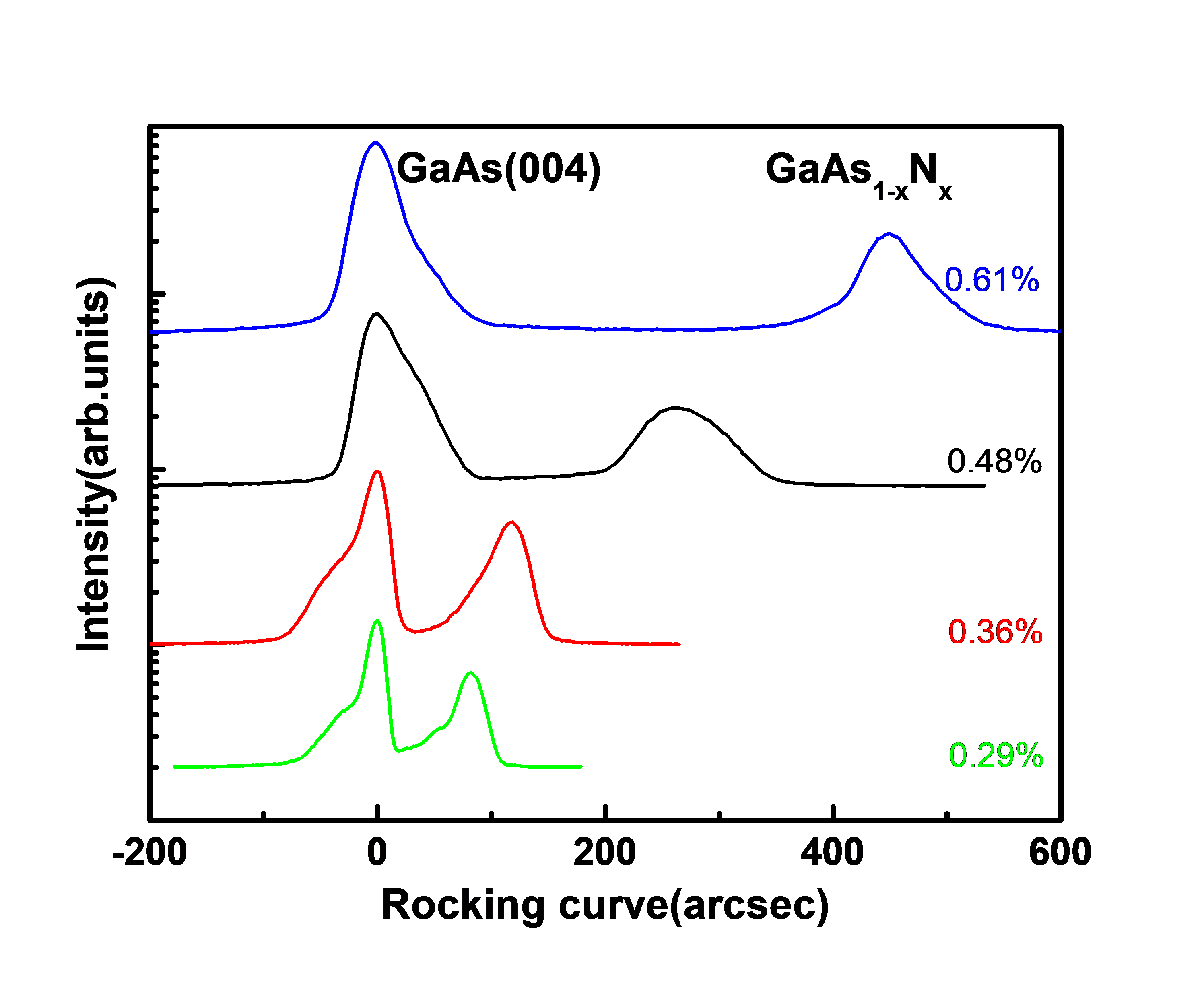##### (1)
$a_{0}=a\left(1-\frac{C_{11}}{C_{11}+2 C_{12}} · \frac{a_{∥}-a_{\perp}}{a_{∥}}\right)$

where C$_{11}$ and C$_{12}$ are the elastic constants of GaAs$_{\mathrm{1-x}}$N$_{\mathrm{x}}$, which are taken as the nitrogen compositions of weighted mean values between the values of GaAs and β-GaN. The elastic constants for GaAs$_{\mathrm{1-x}}$N$_{\mathrm{x}}$ are calculated from the nitrogen composition using C$_{11}$ = 29.6${\times}$10$^{11}$, C$_{12}$ = 1.54${\times}$10$^{11}$ dyn/cm$^{2}$ for β-GaN and C$_{11}$ = 11.883${\times}$10$^{11}$, C$_{12}$ = 5.383${\times}$10$^{11}$ dyn/ cm$^{2}$ for GaAs. The strain in the growth direction in the GaAs$_{\mathrm{1-x}}$N$_{\mathrm{x}}$ strained layer on GaAs substrate is obtained as

##### (2)
$\varepsilon_{\perp}=\frac{a_{\perp}-a_{0}}{a_{0}}$

Also the in-plane strain in the GaAs$_{\mathrm{1-x}}$N$_{\mathrm{x}}$ strained layer is obtained as

##### (3)
$\varepsilon_{∥}=\frac{a_{∥}-a_{0}}{a_{0}}$

Because a GaAs$_{\mathrm{1-x}}$N$_{\mathrm{x}}$ strained layer grown on a GaAs (001) substrate is under biaxial stress, the in-plane strain $ε_{\parallel }$ is related to $ε_{\bot }$ by

##### (4)
$\varepsilon_{∥}=-\frac{\mathrm{C}_{11}}{\mathrm{C}_{12}} \varepsilon_{\perp}$

The nitrogen composition is determined assuming Vegard's rule,

##### (5)
\begin{aligned} a_{GaAs_{1-x}N_{x}}= &xa_{\beta-GaN}+(1-x)a_{GaAs} \\ &-\theta_{GaAsN}(1-x)x\end{aligned}

##### (7)
$\Delta \omega_{\text {strain }}=\frac{1}{\omega_{0}}\left(q-p \frac{C_{12}}{C_{11}}\right) \dot{O}=b \varepsilon_{\|}$

Fig. 2. Raman spectra of strained GaAs$_{\mathrm{1-x}}$N$_{\mathrm{x}}$ epilayers with of four different nitrogen compositions of 0.29 %, 0.36 %, 0.48 % and 0.61 %.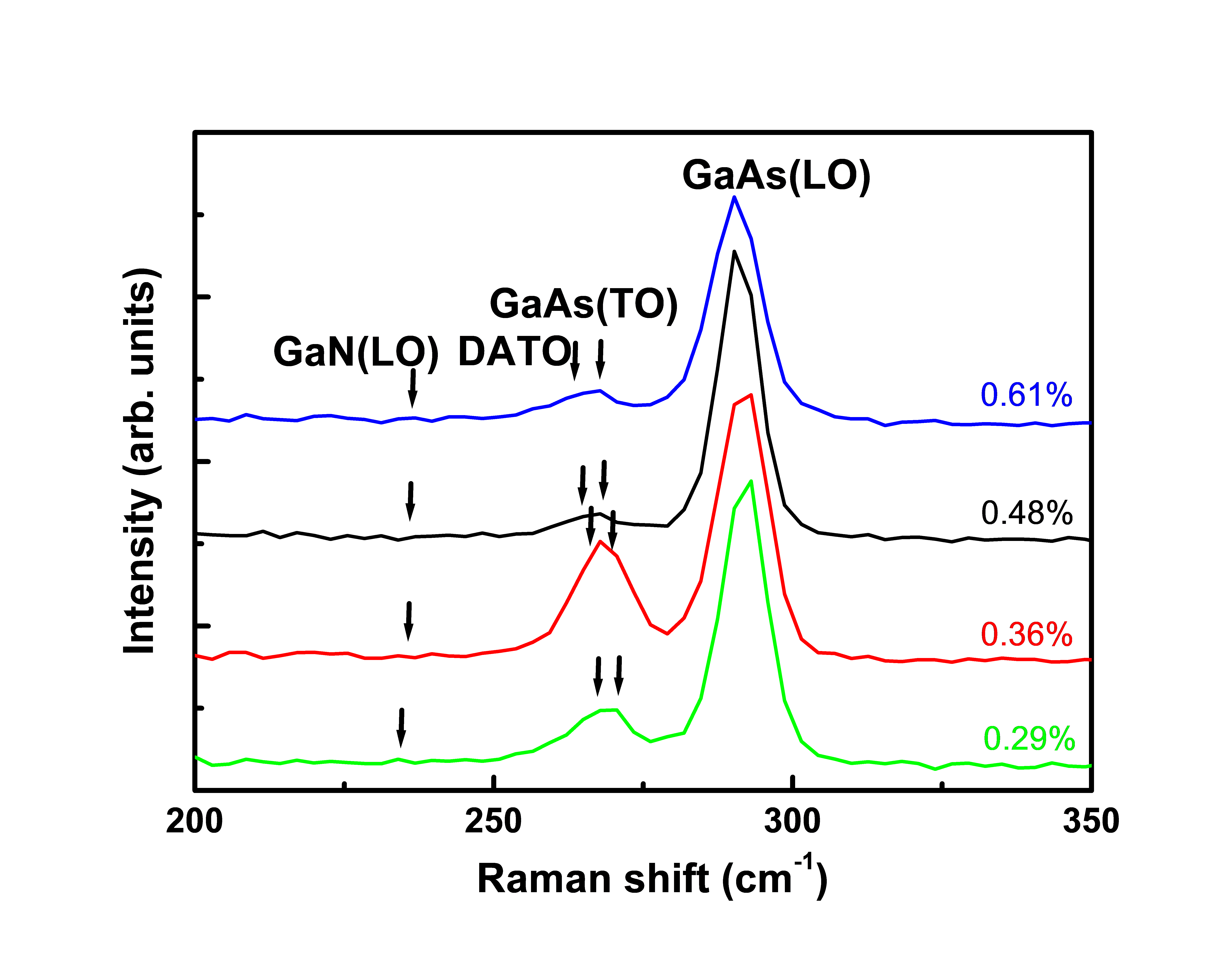where ${ω}$$_{0} is the unstrained Raman frequency, C_{11} and C_{12} are elastic constants, and p and q are phonon deformation parameters. The magnitude of Δω_{\mathrm{alloy}} depends linearly on the nitrogen composition x as it follows the relationship ##### (8) Δω _{\text{alloy}}=ω _{\text{layer}}- ω _{\text{wafer}}=\mathrm{ax}. Fig. 3. Nitrogen compositions dependence of strain of four strained GaAs_{\mathrm{1-x}}N_{\mathrm{x}} epilayers with various nitrogen compositions of 0.29 %, 0.36 %, 0.48 % and 0.61 %, the strain was measured determined HR-XRD and Raman spectroscopy.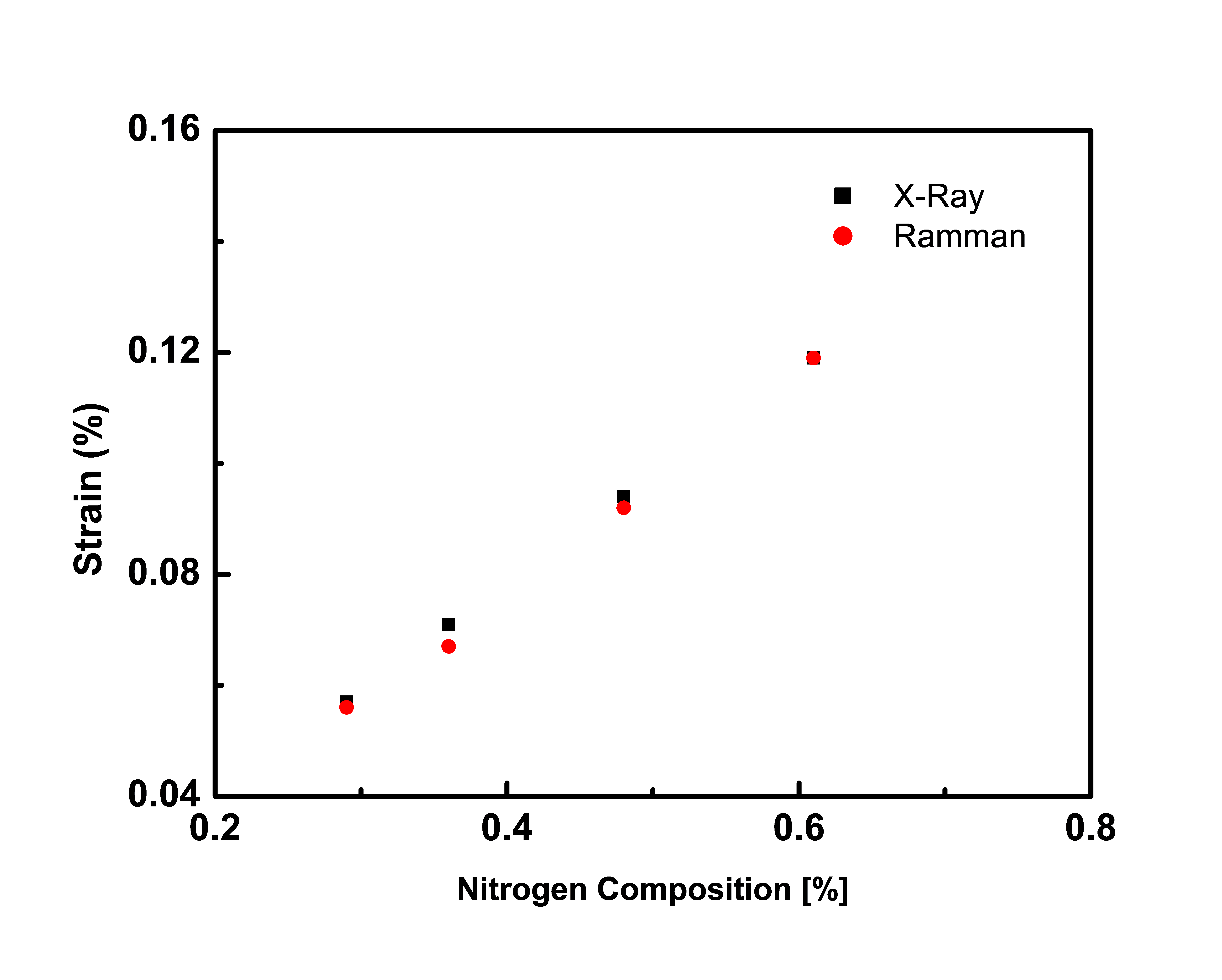The in-plane tensile strain in the strained GaAs_{\mathrm{1-x}}N_{\mathrm{x}} epilayer is calculated from the strain versus Raman spectral peak shift (Δω_{\mathrm{strain}} = {ω}$$_{\mathrm{layer}}$ − ${ω}$$_{\mathrm{wafer}}$) relative to the GaAs wafer. The equation can be simplified to $Δω_{\mathrm{strain}}$ = $bε_{∥}$, where $ε_{∥}$ is the tensile strain and b} = ${-}$ (62.7${\pm}$40) cm$^{-1}$ (19). The strain contribution $Δω_{\mathrm{strain}}$ has to be deduced from the total Raman shift in order to determine the nitrogen composition dependence of the Raman shift. The Raman shift of relaxed GaAs$_{\mathrm{1-x}}$N$_{\mathrm{x}}$ alloys, $Δω_{\mathrm{alloy}}$, can be easily calculated by using Eq (8), where a = ${-}$ (7.54${\pm}$4.0) cm$^{-1}$ (19). The Raman shift of strained GaAs$_{\mathrm{1-x}}$N$_{\mathrm{x}}$ epilayers, $Δω_{\mathrm{alloy}}$, with various nitrogen compositions of 0.29 %, 0.36 %, 0.48 % and 0.61 % are evaluated to be 0.021, 0.027, 0.036 and 0.045 cm$^{{-}1}$, respectively. The estimated value of the Raman shift for strain of strained GaAs$_{\mathrm{1-x}}$N$_{\mathrm{x}}$ epilayers for various nitrogen compositions of 0.29 %, 0.36 %, 0.48 % and 0.61 % are 293.08, 292.34, 290.78, and 289.11 cm$^{{-}1}$, respectively. With Eq (7), the in-plane tensile strains of strained GaAs$_{\mathrm{1-x}}$N$_{\mathrm{x}}$ epilayers for various nitrogen compositions of 0.29 %, 0.36 %, 0.48 % and 0.61 % are of 0.056 %, 0.067%, 0.092% and 0.119%, respectively. This result shows that the in-plane tensile strain of the strained GaAs$_{\mathrm{1-x}}$N$_{\mathrm{x}}$ epilayers increases as the nitrogen composition increases. Fig. 3 show the nitrogen compositions dependence of strain of four strained GaAs$_{\mathrm{1-x}}$N$_{\mathrm{x}}$ epilayers with various nitrogen compositions of 0.29 %, 0.36 %, 0.48 % and 0.61 %, the strain was measured determined HR-XRD and Raman spectroscopy.

Fig. 4. Temperature dependence of the photocurrent spectra of the strained GaAs$_{\mathrm{1-x}}$N$_{\mathrm{x}}$ epilayers with of four different nitrogen compositions of (a) 0.29 %, (b) 0.36 %, (c) 0.48 %, (d) 0.61 %.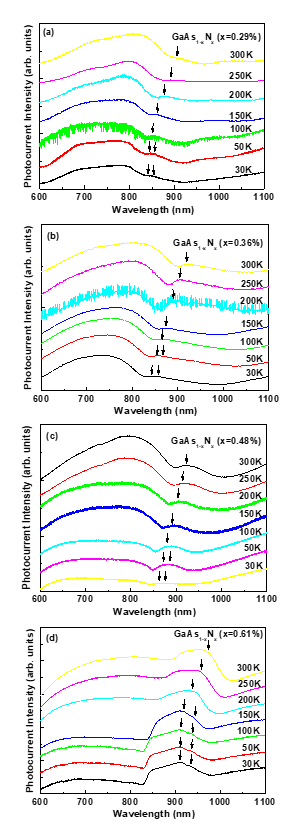The temperature dependences of the photocurrent spectra of strained GaAs$_{\mathrm{1-x}}$N$_{\mathrm{x}}$ epilayers with various nitrogen compositions were measured at temperatures ranging from 300 to 30 K. Fig. 4 show the temperature dependence of photocurrent spectra of four strained GaAs$_{\mathrm{1-x}}$N$_{\mathrm{x}}$ epilayers with various nitrogen compositions of 0.29 %, 0.36 %, 0.48 % and 0.61 %. The wavelength ranges from 600-1100 nm. The photocurrent spectra are dominated by the GaAs$_{\mathrm{1-x}}$N$_{\mathrm{x}}$-related transitions and the GaAs related band edge photocurrent peak seems to be completely dependent on the temperature, as can be clearly seen in Fig. 4. The GaAs$_{\mathrm{1-x}}$N$_{\mathrm{x}}$-related transition photocurrent spectra peaks are found to consist of two bands whose valence-band splitting increase with increasing nitrogen composition. The two features near the GaAs$_{\mathrm{1-x}}$N$_{\mathrm{x}}$-related transition photocurrent peaks in the low-energy peak of the photocurrent spectra of the strained GaAs$_{\mathrm{1-x}}$N$_{\mathrm{x}}$ epilayers are related to the GaAs$_{\mathrm{1-x}}$N$_{\mathrm{x}}$ fundamental energy gap. Epitaxial GaAs$_{\mathrm{1-x}}$N$_{\mathrm{x}}$ epilayers grown on (100) GaAs substrate are under tensile strain. The doublet is the photocurrent peaks of the strained status of the GaAs$_{\mathrm{1-x}}$N$_{\mathrm{x}}$ epilayers. We assign the first and the second photocurrent spectra peaks to the split light-hole(E$_{\mathrm{LH}}$) and heavy-hole (E$_{\mathrm{HH}}$) transitions in the strained GaAs$_{\mathrm{1-x}}$N$_{\mathrm{x}}$ epilayers (21), respectively. This analysis provides us with fine information about the strain status and the composition of the strained GaAs$_{\mathrm{1-x}}$N$_{\mathrm{x}}$ epilayers. The energy gaps associated with the transitions from E$_{\mathrm{HH}}$ and E$_{\mathrm{LH}}$ of valence bands to the conduction band, as well as the valence band splitting ${Δ}$E=E$_{\mathrm{HH}}$ - E$_{\mathrm{LH}}$, can be related to the in-plane strain.

Fig. 4 shows that the photocurrent peak wavelength was red shifted as increasing temperature due to the decreased band gap of the strained GaAs$_{\mathrm{1-x}}$N$_{\mathrm{x}}$ epilayers.

All of the photocurrent spectra peaks are dominated by an intense optical band to band transition that is originated to transition of the valance band electrons. For photocurrent spectra, the low photon energy cut-off of the response spectra was determined by using the band gap energy or the absorption edge of the semiconductor. A low photon energy cut-off was also observed in the response spectra characteristics because at low photon energy, the value of the absorption appear very large in semiconductors and all the incident optical band gap energy is absorbed near the surface. The photocurrent spectra show the valence band splitting peaks at low temperature and their peaks are red-shifted with increasing temperature.

Fig. 5. Temperature dependence of the photocurrent peak energies of the strained GaAs$_{\mathrm{1-x}}$N$_{\mathrm{x}}$ epilayers with of four different nitrogen compositions of 0.29 %, 0.36 %, 0.48 % and 0.61 %.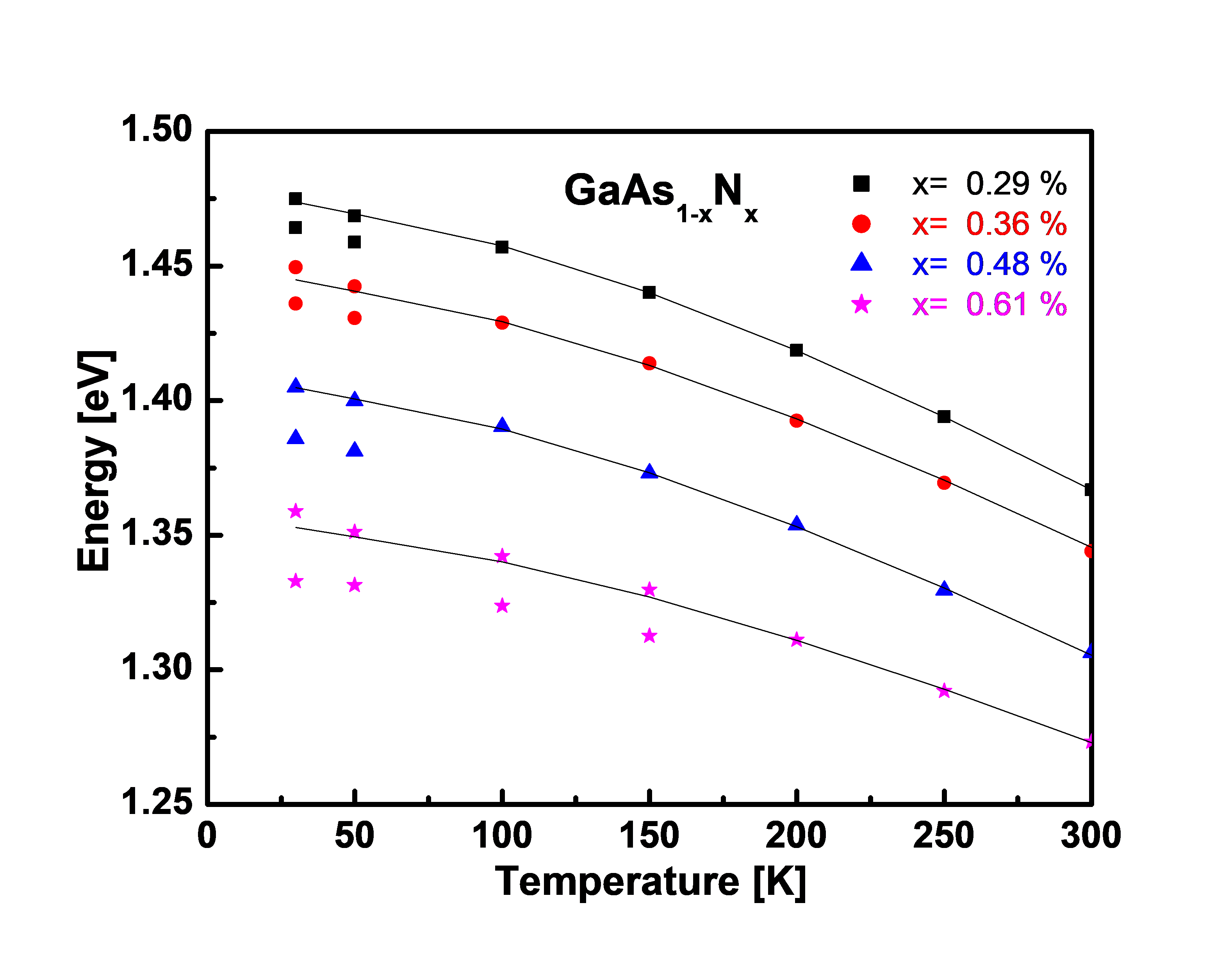Fig. 6. Measured valence band splitting ${Δ}$E=E$_{\mathrm{HH}}$ - E$_{\mathrm{LH}}$ of strained GaAs$_{\mathrm{1-x}}$N$_{\mathrm{x}}$ epilayers versus nitrogen composition dependence.Fig. 5 summarizes the temperature dependence of the GaAs$_{\mathrm{1-x}}$N$_{\mathrm{x}}$-related transition photocurrent peaks (E$_{\mathrm{LH}}$ and E$_{\mathrm{HH}}$) energies of the strained GaAs$_{\mathrm{1-x}}$N$_{\mathrm{x}}$ epilayers with various nitrogen compositions. The temperature dependence of the direct energy band gap can be described by the Bose−Einstein expression proposed by Lautenschlager et al. (22) or the Varshni equation (23). The Varshni relation, E$_{\mathrm{g}}$(T) = E$_{0}$ - αT$^{2}$/(β + T), and the Bose−Einstein expression, E$_{\mathrm{g}}$(T) = E$_{\mathrm{B}}$ − a/[exp(θ/T) - 1], have been used to explain the temperature dependence of the fundamental band gap in semiconductors. E$_{\mathrm{g}}$ and E$_{\mathrm{B}}$ are the band gaps at T = 0 K while α and β are the Varshni coefficients. a is the electron-average phonon coupling constant, and θ corresponds to the average phonon temperature. Both expressions give similarly good fits to the measured E$_{\mathrm{g}}$(T) in GaAs. The parameters fitted for GaAs are E$_{0}$ = 1.519 eV, α = 5.405 ⅹ 10$^{-4}$ eV/K, and β = 204 K, and for GaN, E$_{0}$ = 3.512 eV, α = 5.66 ⅹ 10$^{-4}$ eV/K, and β = 737.9 K in the Varshni relation. For our experimental result, the temperature dependence of the GaAs$_{\mathrm{1-x}}$N$_{\mathrm{x}}$-related transition photocurrent peaks (E$_{\mathrm{LH}}$ and E$_{\mathrm{HH}}$) energies of the strained GaAs$_{\mathrm{1-x}}$N$_{\mathrm{x}}$ epilayer can be described by using Varshni’s empirical expression. As shown in the Fig. 5, the solid lines are fits of the Varshni equations of the measured data for GaAs$_{\mathrm{1-x}}$N$_{\mathrm{x}}$ strained layers with nitrogen composition of 0.29 %, 0.36 %, 0.48 % and 0.61 %, respectively. The fitted parameters for the strained GaAs$_{\mathrm{1-x}}$N$_{\mathrm{x}}$ epilayers with nitrogen composition show the expected trend of decreasing E$_{0}$, α and β as increasing nitrogen composition. For the strained GaAs$_{\mathrm{1-x}}$N$_{\mathrm{x}}$ epilayers with nitrogen composition increase as shown in the Fig. 4 and 5, we observe a significant valence band heavy-holes to conduction and valence band light-holes to conduction transitions due to the strain-induced valence band splitting in the strained GaAs$_{\mathrm{1-x}}$N$_{\mathrm{x}}$ epilayers and due to the lattice mismatch to GaAs substrate. These photocurrent spectra with increasing nitrogen composition, the dominant valence band splitting ${Δ}$E=E$_{\mathrm{HH}}$ - E$_{\mathrm{LH}}$ to the increasing. The measured valence band splitting ${Δ}$E=E$_{\mathrm{HH}}$ - E$_{\mathrm{LH}}$ of the strained GaAs$_{\mathrm{1-x}}$N$_{\mathrm{x}}$ epilayers versus nitrogen composition dependence are shown in Fig. 6. As shown in the Fig. 6, the solid lines are fit the valence band splitting (${Δ}$E) of the measured data for strained GaAs$_{\mathrm{1-x}}$N$_{\mathrm{x}}$ epilayers with nitrogen composition and temperature dependence. The tensile strain is accompanied by splitting of light- and heavy-hole bands as the content of nitrogen composition increases to become 26.1 meV at x=0.61%.

## IV. CONCLUSIONS

We have investigated the Raman spectroscopy and the photocurrent spectra of the strained GaAs$_{\mathrm{1-x}}$N$_{\mathrm{x}}$ epilayers grown on GaAs (001) substrate by using metalorganic chemical vapor deposition with different nitrogen compositions. The nitrogen composition and strain in the strained GaAs$_{\mathrm{1-x}}$N$_{\mathrm{x}}$ epilayers was determined from both X-ray diffraction and Vegard’s rule. The tensile strain of the strained GaAs$_{\mathrm{1-x}}$N$_{\mathrm{x}}$ epilayers increases as the nitrogen composition increases. The Raman spectra are observed to be dominated by the longitudinal optical (LO) phonon mode as the strongest peaks show up around 287~292 cm$^{-1}$. Moreover, the weak and broad peaks features in the range of 265~270 cm$^{-1}$ originate from the peaks of transverse optical (TO) phonon mode. And the Raman peak shifts toward lower wave number with increasing nitrogen compositions, which is indicates the presence of tensile strain in the strained GaAs$_{\mathrm{1-x}}$N$_{\mathrm{x}}$ epilayers. The band gap and the valence-band splitting are obtained from the photocurrent spectra. As nitrogen composition increases, the tensile strain between the GaAs$_{\mathrm{1-x}}$N$_{\mathrm{x}}$ epilayer and GaAs substrate increases while the valence-band splitting obtained from the photocurrent spectra increases.

### REFERENCES

1
Yu H.-C., Dec 2010, Performance Improvement of InGaAsN/GaAs Quantum Well Lasers by Using Trimethylantimony Preflow, Applied Physics Express, Vol. 4, No. 1, pp. 0121032
Kitatani T., Feb 2000, A 1.3-${\mathrm{\mu}}$m GaInNAs/GaAs Single-Quantum-Well Laser Diode with a High Characteristic Temperature over 200 K, Japanese Journal of Applied Physics, Vol. 39, No. 2a, pp. 86-873
Kim T. W., Nov 2014, Impact of growth temperature and substrate orientation on dilute-nitride-antimonide materials grown by MOVPE for multi-junction solar cell application, Journal of Crystal Growth, Vol. 405, No. 1, pp. 87-914
Turcotte S., Jun 2008, Experimental investigation of the variation of the absorption coefficient with nitrogen content in GaAsN and GaInAsN grown on GaAs (001), Journal of Applied Physics, Vol. 104, No. 8, pp. 0835115
Sik J., Jan 2001, Band-gap energies, free carrier effects, and phonon modes in strained GaNAs/GaAs and GaNAs/InAs/GaAs superlattice heterostructures measured by spectroscopic ellipsometry, Journal of Applied Physics, Vol. 89, No. 1, pp. 294-3056
Hassen F., Nov 2015, Optical characterization and carriers transfer between localized and delocalized states in Si-doped GaAsN/GaAs epilayer, Thin Solid Films, Vol. 594, No. 2, pp. 168-1717
Giulotto E., May 2019, Strain related relaxation of the GaAs-like Raman mode selection rules in hydrogenated GaAs1${-}$xNx layers, Journal of Applied Physics, Vol. 125, No. 17, pp. 1757018
Kent P. R. C., Zunger A., Aug 2001, Theory of electronic structure evolution in GaAsN and GaPN alloys, PHYSICAL REVIEW B, Vol. 64, No. 11, pp. 1152089
Lindsay A., O’Reilly E. P., Nov 2004, Unification of the band anticrossing and cluster-state models of dilute nitride semiconductor alloys, PHYSICAL REVIEW LETTERS, Vol. 93, No. 18, pp. 19640210
Wei S.-H., Zunger A., May 1994, Optical properties of zinc-blende semiconductor alloys: Effects of epitaxial strain and atomic ordering, PHYSICAL REVIEW B, Vol. 49, No. 20, pp. 14337-1435111
Ozasa K., Mar 1990, Effect of misfit strain on physical properties of InGaP grown by metalorganic molecular‐beam epitaxy, Journal of Applied Physics, Vol. 68, No. 1, pp. 107-11012
Egorov A. Yu., Jul 2005, Determination of strain-induced valence-band splitting in GaAsN thin films from circularly polarized photoluminescence, Journal of Applied Physics, Vol. 98, No. 1, pp. 01353913
Liu H. F., May 2006, Raman scattering probe of anharmonic effects due to temperature and compositional disorder in GaAs$_{\mathrm{1-x}}$N$_{\mathrm{x}}$, Journal of Applied Physics, Vol. 99, No. 10, pp. 10350314
Lin K. I., Mar 2006, Valence-band splitting in InGaPN: Effects of epitaxial strain and atomic ordering, Journal of Applied Physics, Vol. 99, No. 5, pp. 05610315
Kang S., Feb 2020, Indium Composition Dependence of Raman Spectroscopy and Photocurrent of In$_{\mathrm{x}}$Ga$_{\mathrm{1{-}x}}$As Strained Layers Grown by Using MOCVD, Journal of the Korean Physical Society, Vol. 76, No. 3, pp. 231-23616
Uesugi K., Mar 1999, Reexamination of N composition dependence of coherently grown GaNAs band gap energy with high-resolution x-ray diffraction mapping measurements, Applied Physics Letters, Vol. 74, No. 9, pp. 1254-125617
Li W., Pessa M., Likonen J., May 2001, Lattice parameter in GaNAs epilayers on GaAs: Deviation from Vegard’s law, Applied Physics Letters, Vol. 78, No. 19, pp. 2864-286618
Klangtakai P., May 2015, Effect of gamma-ray irradiation on structural properties of GaAsN films grown by metal organic vapor phase epitaxy, Journal of Crystal Growth, Vol. 418, No. 15, pp. 145-15219
Groenen J., Jun 1997, Tensile and compressive strain relief in $In_{x}$ $Ga_{1-x}$ As epilayers grown on InP probed by Raman scattering, Journal of Applied Physics, Vol. 82, No. 2, pp. 803-80520
Hern andez S., Jun 2003, Evidence of phosphorus incorporation into InGaAs/InP epilayers after thermal annealing, Journal of Applied Physics, Vol. 93, No. 11, pp. 9019-902321
Geddo M., Feb 2007, Photoreflectance and reflectance investigation of deuterium-irradiated GaAsN, Applied Physics Letters, Vol. 90, No. 9, pp. 09190722
Lautenschlager P., Mar 1987, Interband critical points of GaAs and their temperature dependence, PHYSICAL REVIEW B, Vol. 35, No. 17, pp. 9174-918923
Varshni Y. P., 1967, Temperature dependence of the energy gap in semiconductors, Physica, Vol. 34, No. 1, pp. 149-154## Author

received the B.S., M.S., and Ph.D. degrees in the Department of Physics from Jeononbuk National University, Korea, in 1985, 1988, and 1993, respectively.

In 2001, he joined the Faculty of Semiconductor Physics Research Center, Jeononbuk National University, Korea, where he is currently a Research Professor.

His research interests include optoelectronic device, Ge on Si growth and optical characterization.

received the B.S. in the Department of Physics from Kunsan National University, Korea, in 2021.

He is currently pursuing the Ph.D. degree (Combined Master’s-Doctoral Program) in the School of Semiconductor and Chemical Engi-neering from Jeononbuk National University, Korea.

His research interests include semiconductor and high-power device.

received the B.S., and M.S. degrees in the Department of Physics from Jeononbuk National University, Korea, in 1988, and 1990, respectively, and the Ph.D. degree from the Department of Physics and Astronomy, University of Tennessee, Knoxville, in 2001.

In 2007, he joined the Faculty of Department of Physics, Jeononbuk National University, where he is currently Professor.

His research interests include Oxides and semiconductor materials growth and characterization.

received the B.S. degree in 1984 and the M.S. degree in 1986 from the Department of Materials Science and Engineering, Korea University in Korea.

He received the Ph.D. degree in 1997 from the Department of Materials Science and Engineering, University of Illinois at Urbana-Champaign, USA.

He joined the Electronics and Telecommunications Research Institute (ETRI) as a researcher in 1986, and became a principal researcher in 2000.

He performed many national projects to develop specific semiconductor devices like MESFET, MOSFET, HBT, HEMT using compound semiconductors like GaAs, GaN, and SiGe.

Ever since his movement to Chonbuk National University in 2004, he has been leading Intelligent Semiconductor Research Laboratory (ISRL) as a professor in the Department of Semiconductor Science and Technology.

The ISRL focuses its research area on SiGe, Ge-on-Si, and III-nitrides for the development of new electronic and photonic devices. Meanwhile, he founded a start-up, Sigetronics, Inc., in 2008, in which various specific semiconductor devices including Zener (for GaN-based LEDs), TVS, FRD, SBD, ESD/EMI filters have been developed and commercialized.

received the B.S., M.S., and Ph.D. degrees in the Department of Physics from Jeononbuk National University, Korea, in 1988, 1990, and 1996, respectively.

In 2001, he joined the Faculty of Semiconductor Physics Research Center, Jeononbuk National University, Korea.

Ever since his movement to the Department of Physics in 2020, where he is currently an Invited Professor.

His research interests include optoelectronic device, compound semiconductor growth and optical characterization.